• Slides: 13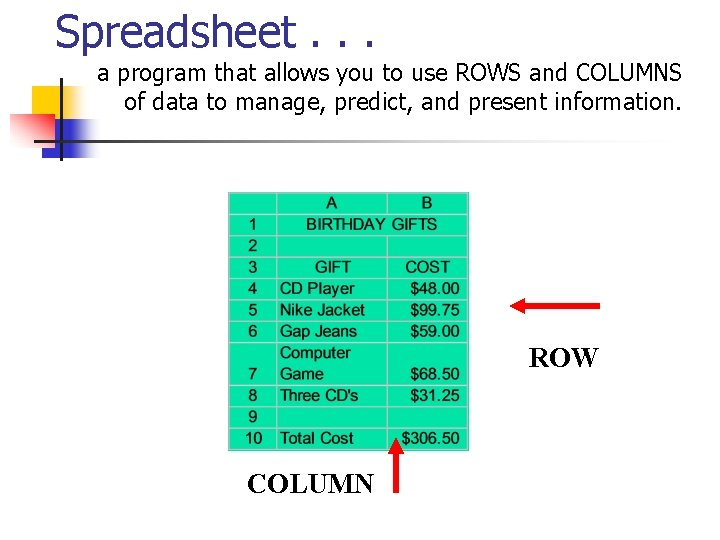Spreadsheet. . . a program that allows you to use ROWS and COLUMNS of data to manage, predict, and present information. ROW COLUMN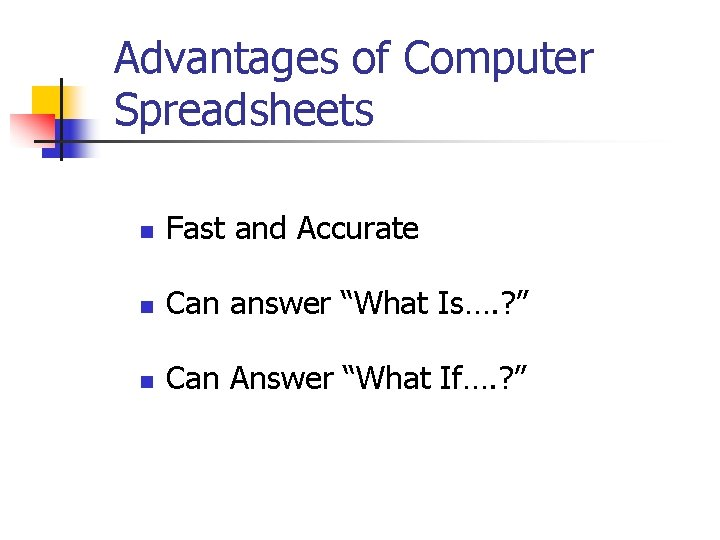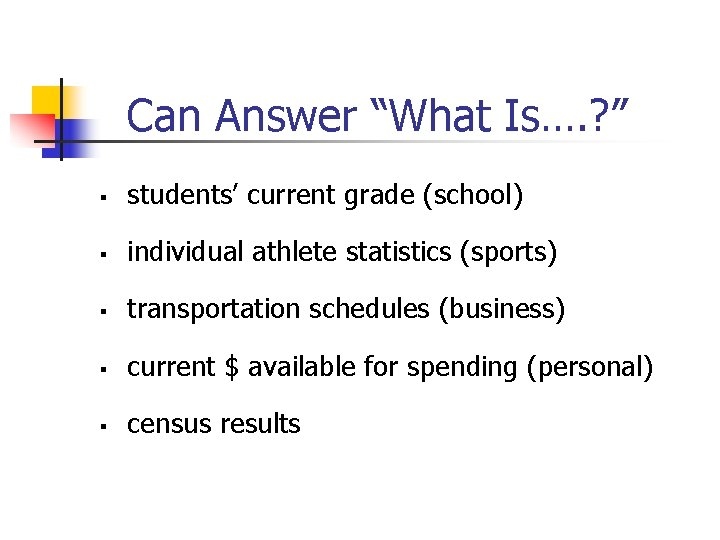Can Answer “What Is…. ? ” § students’ current grade (school) § individual athlete statistics (sports) § transportation schedules (business) § current \$ available for spending (personal) § census results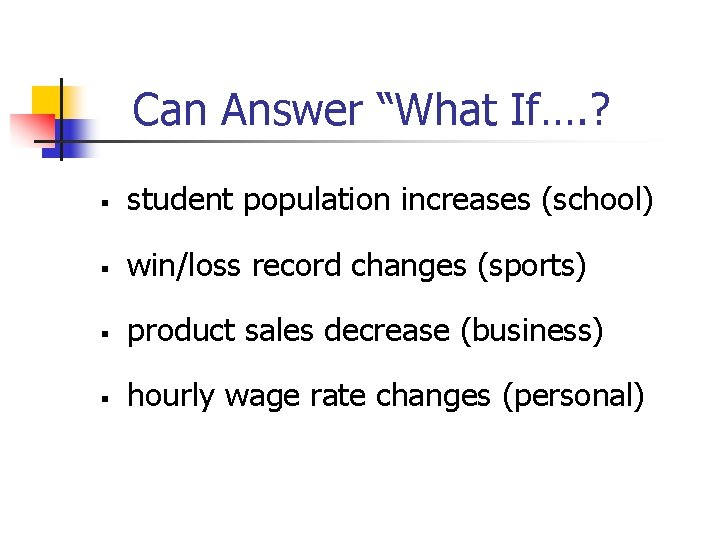Can Answer “What If…. ? § student population increases (school) § win/loss record changes (sports) § product sales decrease (business) § hourly wage rate changes (personal)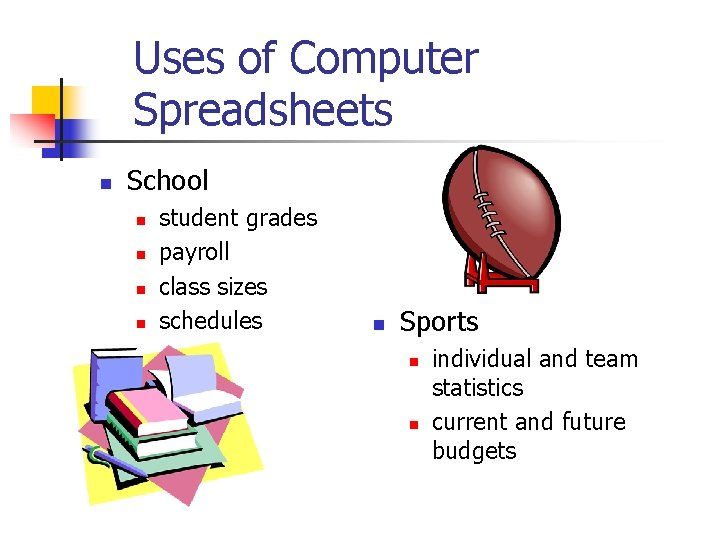Uses of Computer Spreadsheets n School n n student grades payroll class sizes schedules n Sports n n individual and team statistics current and future budgetsUses of Computer Spreadsheets, cont’d n Personal n n checkbook household expenses investments income taxes n Business n n n payroll investments inventory product sales delivery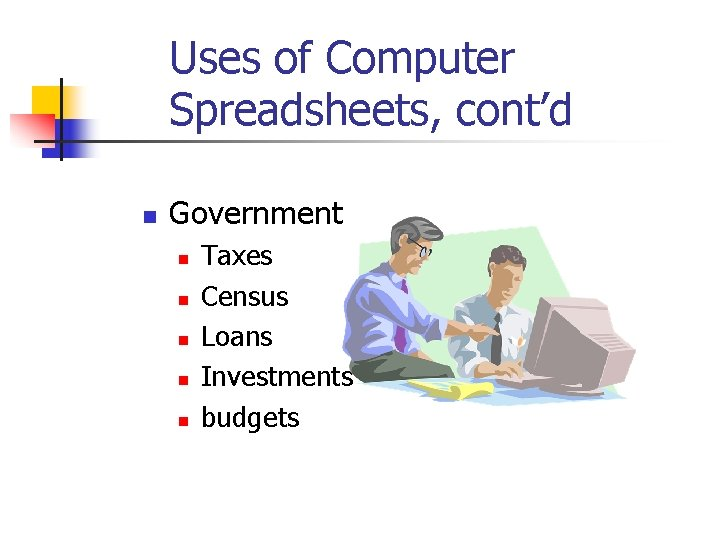Uses of Computer Spreadsheets, cont’d n Government n n n Taxes Census Loans Investments budgets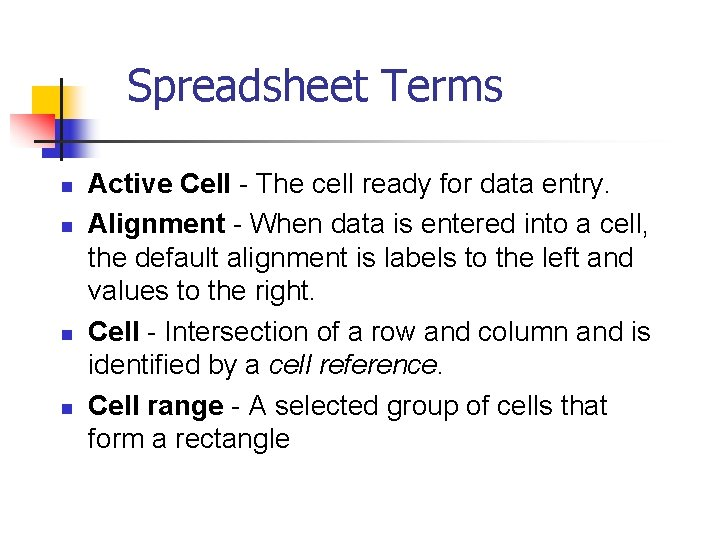Spreadsheet Terms n n Active Cell - The cell ready for data entry. Alignment - When data is entered into a cell, the default alignment is labels to the left and values to the right. Cell - Intersection of a row and column and is identified by a cell reference. Cell range - A selected group of cells that form a rectangleSpreadsheet Terms, cont’d. n n Cell reference - The column letter and the row number. Example: B 12 Column - Identified by letters that appear at the top of the spreadsheet. (Vertical) Formula - Equations with symbols for math operations. Example =B 6+B 7+B 8+B 9 Function - Special formulas that do not use operators to calculate a result. i. e. A shortcut formula. Example: =sum(A 6: A 9)Spreadsheet Terms, cont’d. n n Label - Text, symbols, dates, or numbers not used in calculations. Rows - Identified by numbers on the left side of the spreadsheet. (Horizontal) Spreadsheet - A program that allows you to use rows and columns of data to manage, predict, and present information. Value - A number entered into a spreadsheet cell that will be used for calculations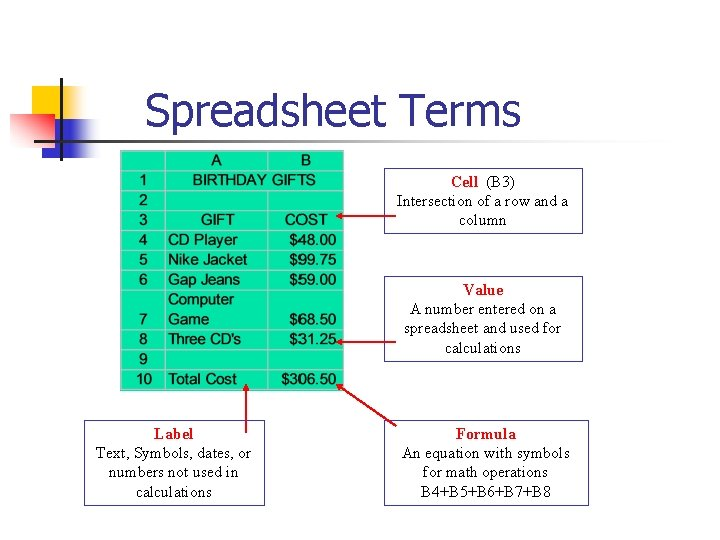Spreadsheet Terms Cell (B 3) Intersection of a row and a column Value A number entered on a spreadsheet and used for calculations Label Text, Symbols, dates, or numbers not used in calculations Formula An equation with symbols for math operations B 4+B 5+B 6+B 7+B 8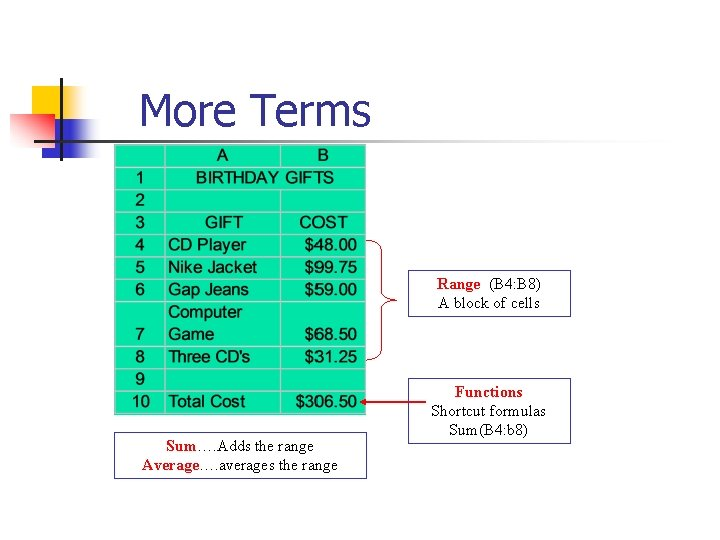More Terms Range (B 4: B 8) A block of cells Sum…. Adds the range Average…. averages the range Functions Shortcut formulas Sum(B 4: b 8)